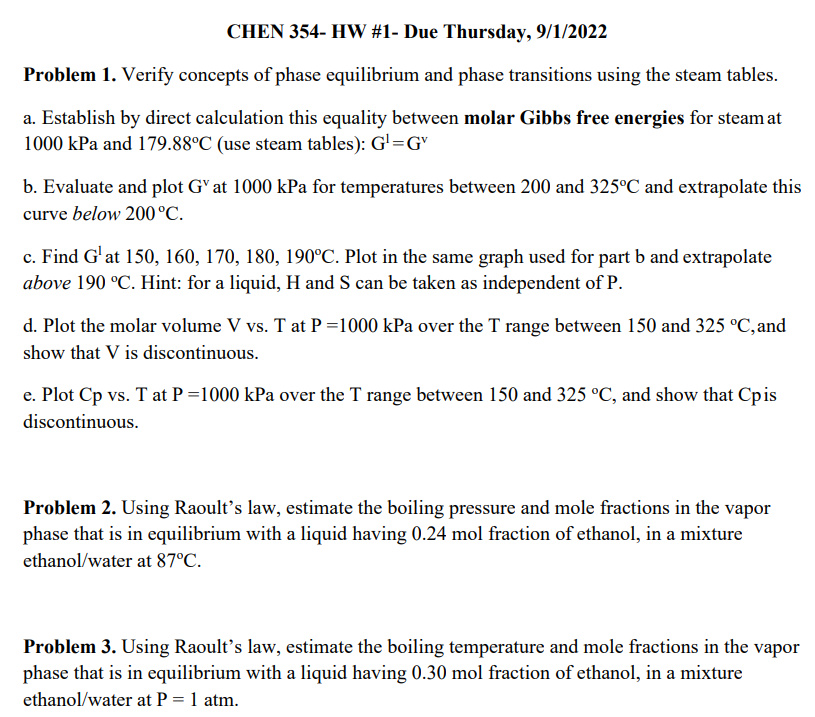# (Solved): please answer all parts of problem 1 Problem 1. Verify concepts of phase equilibrium and phase tra ...Problem 1. Verify concepts of phase equilibrium and phase transitions using the steam tables. a. Establish by direct calculation this equality between molar Gibbs free energies for steam at $$1000 \mathrm{kPa}$$ and $$179.88^{\circ} \mathrm{C}$$ (use steam tables): $$\mathrm{G}^{1}=\mathrm{G}^{\mathrm{v}}$$ b. Evaluate and plot $$\mathrm{G}^{\mathrm{v}}$$ at $$1000 \mathrm{kPa}$$ for temperatures between 200 and $$325^{\circ} \mathrm{C}$$ and extrapolate this curve below $$200^{\circ} \mathrm{C}$$. c. Find $$\mathrm{G}^{1}$$ at $$150,160,170,180,190^{\circ} \mathrm{C}$$. Plot in the same graph used for part $$\mathrm{b}$$ and extrapolate above $$190^{\circ} \mathrm{C}$$. Hint: for a liquid, $$\mathrm{H}$$ and $$\mathrm{S}$$ can be taken as independent of $$\mathrm{P}$$. d. Plot the molar volume $$\mathrm{V}$$ vs. $$\mathrm{T}$$ at $$\mathrm{P}=1000 \mathrm{kPa}$$ over the $$\mathrm{T}$$ range between 150 and $$325^{\circ} \mathrm{C}$$, and show that $$\mathrm{V}$$ is discontinuous. e. Plot $$\mathrm{Cp}$$ vs. T at $$\mathrm{P}=1000 \mathrm{kPa}$$ over the T range between 150 and $$325^{\circ} \mathrm{C}$$, and show that $$\mathrm{Cp}$$ is discontinuous. Problem 2. Using Raoult's law, estimate the boiling pressure and mole fractions in the vapor phase that is in equilibrium with a liquid having $$0.24 \mathrm{~mol}$$ fraction of ethanol, in a mixture ethanol/water at $$87^{\circ} \mathrm{C}$$. Problem 3. Using Raoult's law, estimate the boiling temperature and mole fractions in the vapor phase that is in equilibrium with a liquid having $$0.30 \mathrm{~mol}$$ fraction of ethanol, in a mixture ethanol/water at $$\mathrm{P}=1 \mathrm{~atm}$$.